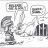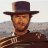# RSI in Laguerre Time with Fractal Energy - Momentum/Trend Indicator

B

#### bubz

##### New member
VIP
@marine Thanks I will try that out.

•J007RMC
M

#### marine

##### New member
•J007RMC
H

#### hjc5812

##### New member
VIP
@hjc5812 2 things, get my original study from page 1 of this post and be sure to save the chart. If you still have trouble, please call this help number: 800-672-2098. Good luck. I'm not here often but someone can help further if you still need it.
Yeah it didn't work. I will have to call to figure this out.
Funny "Trend Momentun and Cycle Trading System" gives me the same problem.

Last edited:
H

#### hjc5812

##### New member
VIP
Sorry guys if this was already answered, but would it be possible to add up and down arrow on the upper chart when the Laguerre RSI crosses oversold and overbought line?

B

#### BWiz

##### New member
Sorry guys if this was already answered, but would it be possible to add up and down arrow on the upper chart when the Laguerre RSI crosses oversold and overbought line?

Hello HJC, Here is the coding for what you are wanting. I also added inputs for oversold and overbought levels for your choosing, so that you can choose when an arrow will print. I personally use a crossing of .2 for OB and a crossing of .8 for OS to generate the arrows due to a more reliable trade entry.

Code:
``````# TheoTrade RSI in Laguerre Time Self Adjusting With Fractal Energy
# Mobius
# V03.06.15.2016
#Bwiz modified to paint arrows and adjust OB_OS values.
#04_26_20

#Inputs:
input nFE = 13;#hint nFE: length for Fractal Energy calculation.

# Variables:
def o;
def h;
def l;
def c;
def CU1;
def CU2;
def CU;
def CD1;
def CD2;
def CD;
def L0;
def L1;
def L2;
def L3;
def RSI;
input OS = .2;
input OB = .8;

# Calculations
o = (open + close) / 2;
h = Max(high, close);
l = Min(low, close);
c = (o + h + l + close) / 4;
def gamma = Log(Sum((Max(high, close) - Min(low, close)), nFE) /
(Highest(high, nFE) - Lowest(low, nFE)))
/ Log(nFE);

L0 = (1 – gamma) * c + gamma * L0;
L1 = -gamma * L0 + L0 + gamma * L1;
L2 = -gamma * L1 + L1 + gamma * L2;
L3 = -gamma * L2 + L2 + gamma * L3;
if L0 >= L1
then {
CU1 = L0 - L1;
CD1 = 0;
} else {
CD1 = L1 - L0;
CU1 = 0;
}
if L1 >= L2
then {
CU2 = CU1 + L1 - L2;
CD2 = CD1;
} else {
CD2 = CD1 + L2 - L1;
CU2 = CU1;
}
if L2 >= L3
then {
CU = CU2 + L2 - L3;
CD = CD2;
} else {
CU = CU2;
CD = CD2 + L3 - L2;
}

RSI = if CU + CD <> 0 then CU / (CU + CD) else 0;

plot isAbove = RSI crosses above OS;
plot isBelow = RSI crosses below OB;
isAbove.SetPaintingStrategy(PaintingStrategy.BOOLEAN_ARROW_UP);
isBelow.SetPaintingStrategy(PaintingStrategy.BOOLEAN_ARROW_DOWN);

# End Code RSI_Laguerre Self Adjusting with Fractal Energy``````

Last edited:
••markos and hjc5812
J

#### J-Fearless

##### New member
Hi @J007RMC

Firstly I'd like to thank you for posting what seems to be an excellent study. I had one or two quick questions if you can spare the time:

1. Do the arrows repaint?
2. What time frame do you think this offers better trades on? Personally I'm finding the 1h20d seems to be great - any thoughts?
3. How are you getting out of trouble - what indicates a false entry signal while using this study?

- J-Fearless

M

#### mansor

##### Member
Guys - This thread has too many pages to go through, and too many variations of the script. Can anyone please advice which version works for 1min1day with accuracy. Thank you all in advance!

B

#### bubz

##### New member
VIP
Use the tick chart one so it can work for Ticks and time frames. But def read up on what FE is for, it does not predict direction just remember that. I think you have to try a few to get one that works for you.

••markos and mansor
B

#### BWiz

##### New member
Guys - This thread has too many pages to go through, and too many variations of the script. Can anyone please advice which version works for 1min1day with accuracy. Thank you all in advance!
Hey Mansor,
I personally use the RSI-L with FE that Mobius made for Theotrade. I use it to trade NQ and ES futures intraday on a 2min time frame to enter and exit trades. I also use an anchor chart of 5-10 times higher time frame so that I am trading with the trend. If the longer time frame is heading up then I only take long entries and vice versa for shorts. You can make small scalps trading against the trend using this indicator, but I dont like doing that unless price action tells me otherwise. Also, I dont find fractal energy of much use on the 2min time frame, its really only useful on longer time frames in my opinion.

•surferdude, markos and mansor
M

#### manishji

##### New member
@J007RMC Very nice... Is there a scan for this study? I get following error when i try to edit scan. The complexity of the expression suggests that it may not be reliable with real-time data.

H

#### hjc5812

##### New member
VIP
@BWiz This is exactly what I was looking for thank a lot!

•BWiz
M

#### mansor

##### Member
Some scripts click right away & some don't. This one gives good entry signals for me on 1m1d. Thank you

•markos and BWiz
J

#### J-Fearless

##### New member
Hi @J007RMC

Is there a a separate thread for this? I have questions which I'm sure would already have been answered if there is.

Thanks
-J

J

#### J-Fearless

##### New member
Hi @J007RMC

Is there a a separate thread for this? I have questions which I'm sure would already have been answered if there is.

Thanks
-J
@J007RMC - it didn't quote the original reply for some reason - I'm referring to RSI_Laguerre_Lines_wTargets

M

#### martyrjohn

##### Member
Is it possible to add trend arrows that appear when the Rsi Laguerre is hooking up and the fractal energy is about to start trending?#### Prison Mike

##### Member
IDK if this will be useful, what can be done with it or even if the code is correct but I pieced together a Fractal Energy based off RSI rather than close.

Genius or Dumb?

Code:
``````declare lower;

#Inputs:
input nFE = 8;#hint nFE: length for Fractal Energy calculation.
input Glength  = 13;
input length = 14;

input price = close;
input averageType = AverageType.WILDERS;
input showBreakoutSignals = no;

def NetChgAvg = MovingAverage(averageType, price - price, length);
def TotChgAvg = MovingAverage(averageType, AbsValue(price - price), length);
def ChgRatio = if TotChgAvg != 0 then NetChgAvg / TotChgAvg else 0;

def data = chgratio;

def w = (2 * Double.Pi / Glength);
def beta = (1 - Cos(w)) / (Power(1.414, 2.0 / betaDev) - 1 );
def alpha = (-beta + Sqrt(beta * beta + 2 * beta));
def Go = Power(alpha, 4) * open +
4 * (1 – alpha) * Go – 6 * Power( 1 - alpha, 2 ) * Go +
4 * Power( 1 - alpha, 3 ) * Go - Power( 1 - alpha, 4 ) * Go;
def Gh = Power(alpha, 4) * high +
4 * (1 – alpha) * Gh – 6 * Power( 1 - alpha, 2 ) * Gh +
4 * Power( 1 - alpha, 3 ) * Gh - Power( 1 - alpha, 4 ) * Gh;
def Gl = Power(alpha, 4) * low +
4 * (1 – alpha) * Gl – 6 * Power( 1 - alpha, 2 ) * Gl +
4 * Power( 1 - alpha, 3 ) * Gl - Power( 1 - alpha, 4 ) * Gl;
def Gc = Power(alpha, 4) * data +
4 * (1 – alpha) * Gc – 6 * Power( 1 - alpha, 2 ) * Gc +
4 * Power( 1 - alpha, 3 ) * Gc - Power( 1 - alpha, 4 ) * Gc;
# Variables:
def o;
def h;
def l;
def c;
def CU1;
def CU2;
def CU;
def CD1;
def CD2;
def CD;
def L0;
def L1;
def L2;
def L3;

# Calculations
o = (Go + Gc) / 2;
h = Max(Gh, Gc);
l = Min(Gl, Gc);
c = (o + h + l + Gc) / 4;
plot gamma =2* (Log(Sum((Max(Gh, Gc) - Min(Gl, Gc)), nFE) /
(Highest(Gh, nFE) - Lowest(Gl, nFE)))
/ Log(nFE));
gamma.SetDefaultColor(Color.YELLOW);
L0 = (1 – gamma) * Gc + gamma * L0;
L1 = -gamma * L0 + L0 + gamma * L1;
L2 = -gamma * L1 + L1 + gamma * L2;
L3 = -gamma * L2 + L2 + gamma * L3;
if L0 >= L1
then {
CU1 = L0 - L1;
CD1 = 0;
} else {
CD1 = L1 - L0;
CU1 = 0;
}
if L1 >= L2
then {
CU2 = CU1 + L1 - L2;
CD2 = CD1;
} else {
CD2 = CD1 + L2 - L1;
CU2 = CU1;
}
if L2 >= L3
then {
CU = CU2 + L2 - L3;
CD = CD2;
} else {
CU = CU2;
CD = CD2 + L3 - L2;
}``````

M

#### martyrjohn

##### Member
Is there a way to make an up arrow and scanner on a chart when the RSI line is hooking out of oversold territory and the fractal is starting to go up?#### markos

##### Well-known member
VIP
Is there a way to make an up arrow and scanner on a chart when the RSI line is hooking out of oversold territory and the fractal is starting to go up?
@martyrjohn add this to the bottom of your study. That's the arrow part of the study. Someone else will have to assist w scan for you, unless you find the scans that exist in other threads. There are a few...
I would suggest that you read some of the threads below. Fractal Energy doesn't move up when the RSI Laguerre is moving up generally. At least I've never seen it. Also suggest that you ignore FE as it is only a signal of when NOT to trade vs when to trade, It's all spelled out in the threads below and in the Tutorial section.

plot isAbove = RSI crosses above OS;
plot isBelow = RSI crosses below OB;
isAbove.SetPaintingStrategy(PaintingStrategy.BOOLEAN_ARROW_UP);
isBelow.SetPaintingStrategy(PaintingStrategy.BOOLEAN_ARROW_DOWN);

•martyrjohn#### markos

##### Well-known member
VIP
No Good @Prison Mike That is a study of the Wilders RSI of the RSI in Laguerre Time study. I could be wrong but Laguerre Time Transform Polynomials are for price. They self adjust with the speed of the stock.#### markos

##### Well-known member
VIP
@J007RMC - it didn't quote the original reply for some reason - I'm referring to RSI_Laguerre_Lines_wTargets
@J-Fearless Check the Tutorials and the similar threads at the page bottom.RSI in Laguerre Time Self Adjusting With Fractal Energy Usage Notes Tutorials 50Need help with TTM Trend and RSI Laguerre strategy Questions 5TMO or RSI in Laguerre Time with FE - Q&A Tutorials 26